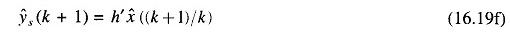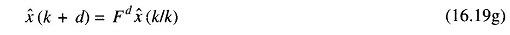## Kalman Filtering Approach:

The time series approach has been widely employed in dealing with the load forecasting problem in view of the relative simplicity of the model forms. However, this method tends to ignore the statistical information about the load data which may often be available and may lead to improved load forecasts if utilized properly. In ARMA model, the model identification problem is not that simple. These difficulties may be avoided in some situations if the Kalman Filtering Approach are utilized.

### Application to Short-term Forecasting

An application of the Kalman filtering algorithm to the load forecasting problem has been first suggested by Toyada et. al. for the very short-term and short-term situations. For the latter case, for example, it is possible to make use of intuitive reasonings to suggest that an acceptable model for load demand would have the form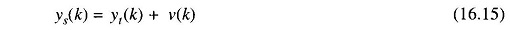where ys(k) is the observed value of the stochastic load at time k, yt(k) is the true value of this load and v(k) is the error in the observed load. In addition, the dynamics of the true load may be expressed as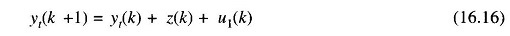where z(k) represents the increment of the load demand at time k and u1(k) represents a disturbance term which accounts for the stochastic perturbations in yt(k). The incremental load itself is assumed to remain constant on an average at every time point and is modelled by the equation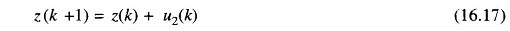where the term u2(k) represents a stochastic disturbance term.

In order to make use of the Kalman Filtering Approach, the noise terms u1(k), u2(k) and ν(k) are assumed to be zero mean independent white Gaussian sequences. Also, the model equations are rewritten in the form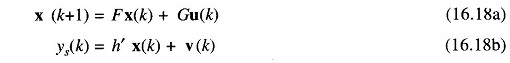where the vectors x(k) and u(k) are defined as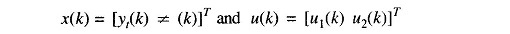The matrices F, G and h’ are then obtained from Eqs. (16.15)-(16.17) easily and have the following values.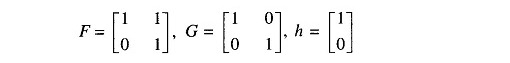Based on model (16.18), it is possible to make use of the Kalman filtering algorithm to obtain the minimum variance estimate of the vector x(k) based on the data ys(k): {ys(1), ys(2)…. ys(k)}. This algorithm consists of the following equations.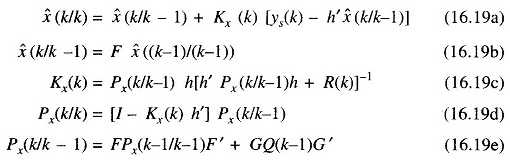where

• Q(k) = covariance of u(k)
• R(k) = covariance of ν(k)
• x̂(k/k) = filtered estimate of x(k)
• x̂(k/k-1) = single step prediction of x(k)
• Kx(k) = filter gain vector of same dimension as x(k)
• Px(k/k) = filtering error covariance
• Px(k/k-1) = prediction error covariance

From Eq. (16.18b) obtain the prediction x̂((k +1)/k)